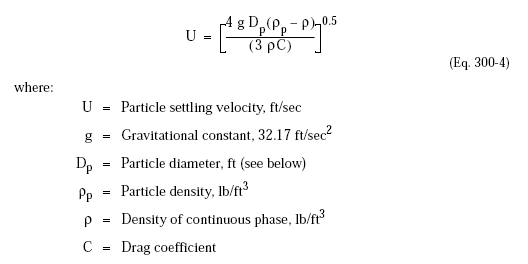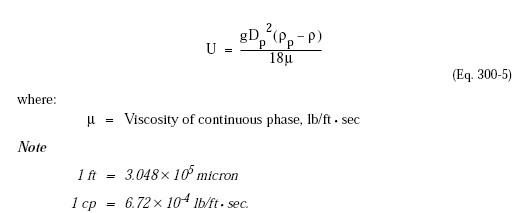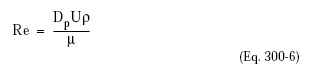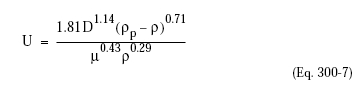﻿ Terminal Velocity – Oil & Gas Industry Technology Updates

## Terminal Velocity

Sizing of vessels for separation of liquid phases is based on a theoretical model and equations for terminal or free-settling velocity of a spherical particle in a fluid. As in vapor-liquid separation, the point is to reduce the velocity of the bulk fluid enough to allow entrained droplets to settle. The general equation is:In the Stokes Law or “laminar flow” region (Reynolds number <= 2), the above equation reduces to:The Reynolds number, Re, uses the particle (not the vessel) diameter:In the “intermediate flow” region (2 < Reynolds number <= 1000), use the following:Settling of liquid droplets within another liquid is usually within the laminar flow region. Assume laminar flow and use Equation 300-5. Compute U for a desired Dp or Dp for a known U as required. Then use Equation 300-6 to compute Re; if it is greater than 2, use Equation 300-7. Reminder: this has nothing to do with movement of bulk phases within the vessel, which is assumed to be in plug flow.